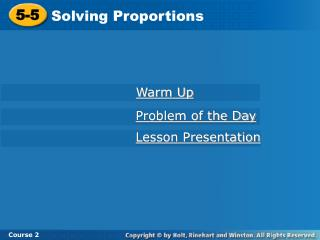DownloadDownload Presentation5-5

# 5-5

Télécharger la présentation## 5-5

- - - - - - - - - - - - - - - - - - - - - - - - - - - E N D - - - - - - - - - - - - - - - - - - - - - - - - - - -
##### Presentation Transcript

1. 5-5 Solving Proportions Course 2 Warm Up Problem of the Day Lesson Presentation

2. 5-5 Solving Proportions Course 2 Warm Up Determine whether the ratios are proportional. 5 8 15 24 , 1. yes 16 25 12 15 , 2. no 15 10 20 16 , 3. no 14 18 42 54 , yes 4.

3. 5-5 Solving Proportions Course 2 Learn to solve proportions by using cross products.

4. 5-5 Solving Proportions Course 2 Insert Lesson Title Here Vocabulary cross product

5. 5-5 Solving Proportions 6 15 2 5 = Course 2 For two ratios, the product of the numerator in one ratio and the denominator in the other is a cross product. If the cross products of the ratios are equal, then the ratios form a proportion. 5 · 6 = 30 2 · 15 = 30

6. 5-5 Solving Proportions CROSS PRODUCT RULE a b c d In the proportion = , the cross products, a · d and b · c are equal. Course 2 You can use the cross product rule to solve proportions with variables.

7. 5-5 Solving Proportions 9 15 m 5 = Course 2 Additional Example 1: Solving Proportions Using Cross Products Use cross products to solve the proportion. 15 · m = 9 · 5 The cross products are equal. 15m = 45 Multiply. 15m 15 = 45 15 Divide each side by 15 to isolate the variable. m = 3

8. 5-5 Solving Proportions 6 7 m 14 = Course 2 Insert Lesson Title Here Check It Out: Example 1 Use cross products to solve the proportion. 7 · m = 6 · 14 The cross products are equal. 7m = 84 Multiply. 7m 7 84 7 Divide each side by 7 to isolate the variable. = m = 12

9. 5-5 Solving Proportions Course 2 When setting up a proportion to solve a problem, use a variable to represent the number you want to find. In proportions that include different units of measurement, the units in the numerators must be the same and the units in the denominators must be the same. 16 mi 4 hr 8 mi x hr =

10. 5-5 Solving Proportions 1 Understand the Problem Course 2 Additional Example 2:Problem Solving Application If 3 volumes of Jennifer’s encyclopedia takes up 4 inches of space on her shelf, how much space will she need for all 26 volumes? Rewrite the question as a statement. • Find the space needed for 26 volumes of the encyclopedia. List the important information: • 3 volumes of the encyclopedia take up 4 inches of space.

11. 5-5 Solving Proportions Make a Plan 2 Course 2 Additional Example 2 Continued Set up a proportion using the given information. Let x represent the inches of space needed. volumes inches 3 volumes 4 inches 26 volumes x =

12. 5-5 Solving Proportions 3 Solve Course 2 Additional Example 2 Continued 3 4 26 x Write the proportion. = 3 · x = 4 · 26 The cross products are equal. 3x = 104 Multiply. Divide each side by 3 to isolate the variable. 3x 3 104 3 = 2 3 x = 34 2 3 She needs 34 inches for all 26 volumes.

13. 5-5 Solving Proportions 4 Course 2 Additional Example 2 Continued Look Back 4 · 26 = 104 26 3 4 = 2 3 34 2 3 = 104 3 · 34 2 3 is the The cross products are equal, so 34 answer

14. 5-5 Solving Proportions 1 Understand the Problem Course 2 Check It Out: Example 2 John filled his new radiator with 6 pints of coolant, which is the 10 inch mark. How many pints of coolant would be needed to fill the radiator to the 25 inch level? Rewrite the question as a statement. • Find the number of pints of coolant required to raise the level to the 25 inch level. List the important information: • 6 pints is the 10 inch mark.

15. 5-5 Solving Proportions Make a Plan 2 Course 2 Check It Out: Example 2 Continued Set up a proportion using the given information. Let p represent the pints of coolant. 6 pints 10 inches p 25 inches pints inches =

16. 5-5 Solving Proportions 3 Solve Course 2 Check It Out: Example 2 Continued 6 10 p 25 Write the proportion. = 10 · x = 6 · 25 The cross products are equal. 10x = 150 Multiply. Divide each side by 10 to isolate the variable. 10x 10 150 10 = p = 15 15 pints of coolant will fill the radiator to the 25 inch level.

17. 5-5 Solving Proportions 4 Course 2 Check It Out: Example 2 Continued Look Back 10 · 15 = 150 15 6 10 = 25 6 · 25 = 150 The cross products are equal, so 15 is the answer.

18. 5-5 Solving Proportions x 9 19 57 = 2. Course 2 Insert Lesson Title Here Lesson Quiz: Part I Use cross products to solve the proportion. 45 t 25 20 1. = t = 36 x = 3 2 3 r 36 = 3. r = 24

19. 5-5 Solving Proportions Course 2 Insert Lesson Title Here Lesson Quiz: Part II 4. Carmen bought 3 pounds of bananas for \$1.08. June paid \$1.80 for her purchase of bananas. If they paid the same price per pound, how many pounds did June buy? 5 pounds